# 1 Introduction

A sequence logo, based on information theory, has been widely used as a graphical representation of sequence conservation (aka motif) in multiple amino acid or nucleic acid sequences. Sequence motif represents conserved characteristics such as DNA binding sites, where transcription factors bind, and catalytic sites in enzymes. Although many tools, such as seqlogo, have been developed to create sequence motif and to represent it as individual sequence logo, software tools for depicting the relationship among multiple sequence motifs are still lacking. We developed a flexible and powerful open-source R/Bioconductor package, motifStack, for visualization of the alignment of multiple sequence motifs.

# 2 Prepare environment

You will need ghostscript: the full path to the executable can be set by the environment variable R_GSCMD. If this is unset, a GhostScript executable will be searched by name on your path. For example, on a Unix, linux or Mac “gs” is used for searching, and on Windows the setting of the environment variable GSC is used, otherwise commands “gswi64c.exe” then “gswin32c.exe” are tried.

Example on Windows: assume that the gswin32c.exe is installed at C:\ Program Files\ gs\ gs9.06\ bin, then open R and try:

Sys.setenv(R_GSCMD=file.path("C:", "Program Files", "gs",
"gs9.06", "bin", "gswin32c.exe"))

# 3 Examples of using motifStack

## 3.1 plot a DNA sequence logo with different fonts and colors

Users can select different fonts and colors to draw the sequence logo.

suppressPackageStartupMessages(library(motifStack))
"extdata", "bin_SOLEXA.pcm"))
pcm <- pcm[,3:ncol(pcm)]
rownames(pcm) <- c("A","C","G","T")
motif <- new("pcm", mat=as.matrix(pcm), name="bin_SOLEXA")
##pfm object
#motif <- pcm2pfm(pcm)
#motif <- new("pfm", mat=motif, name="bin_SOLEXA")
opar<-par(mfrow=c(4,1))
plot(motif)
#plot the logo with same height
plot(motif, ic.scale=FALSE, ylab="probability")
#try a different font
plot(motif, font="mono,Courier")
#try a different font and a different color group
motif@color <- colorset(colorScheme='basepairing')
plot(motif,font="Times")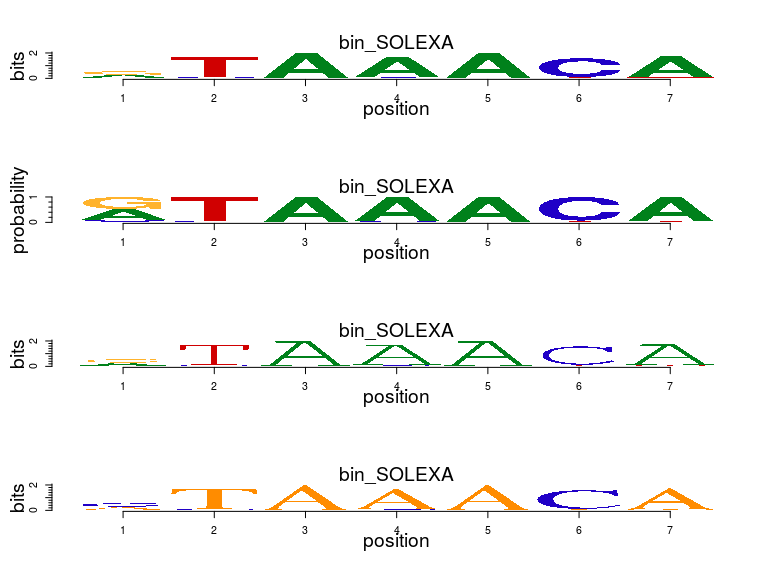par(opar)

## 3.4 plot sequence logo stack

motifStack is designed to show multiple motifs in same canvas. To show the sequence logo stack, the distance of motifs need to be calculated first for example by using MotIV::motifDistances, which implemented STAMP. After alignment, users can use plotMotifLogoStack function to draw sequence logos stack or use plotMotifLogoStackWithTree function to show the distance tree with the sequence logos stack or use plotMotifStackWithRadialPhylog function to plot sequence logo stack in radial style in the same canvas. There is a shortcut function named as motifStack. Use stack layout to call plotMotifLogoStack, treeview layout to call plotMotifLogoStackWithTree and radialPhylog to call plotMotifStackWithRadialPhylog.

suppressPackageStartupMessages(library(motifStack))
#####Input#####
pcms<-readPCM(file.path(find.package("motifStack"), "extdata"),"pcm$") motifs<-lapply(pcms,pcm2pfm) ## plot stacks #motifStack(motifs, layout="stack", ncex=1.0) ## plot stacks with hierarchical tree #motifStack(motifs, layout="tree") ## When the number of motifs is too much to be shown in a vertical stack, ## motifStack can draw them in a radial style. ## random sample from MotifDb library("MotifDb") ## See system.file("LICENSE", package="MotifDb") for use restrictions. matrix.fly <- query(MotifDb, "Dmelanogaster") motifs2 <- as.list(matrix.fly) ## use data from FlyFactorSurvey motifs2 <- motifs2[grepl("Dmelanogaster\\-FlyFactorSurvey\\-", names(motifs2))] ## format the names names(motifs2) <- gsub("Dmelanogaster_FlyFactorSurvey_", "", gsub("_FBgn\\d+$", "",
gsub("[^a-zA-Z0-9]","_",
gsub("(_\\d+)+$", "", names(motifs2))))) motifs2 <- motifs2[unique(names(motifs2))] pfms <- sample(motifs2, 50) ## creat a list of object of pfm motifs2 <- lapply(names(pfms), function(.ele, pfms){new("pfm",mat=pfms[[.ele]], name=.ele)} ,pfms) ## trim the motifs motifs2 <- lapply(motifs2, trimMotif, t=0.4) ## setting colors library(RColorBrewer) color <- brewer.pal(12, "Set3") ## plot logo stack with radial style motifStack(motifs2, layout="radialPhylog", circle=0.3, cleaves = 0.2, clabel.leaves = 0.5, col.bg=rep(color, each=5), col.bg.alpha=0.3, col.leaves=rep(color, each=5), col.inner.label.circle=rep(color, each=5), inner.label.circle.width=0.05, col.outer.label.circle=rep(color, each=5), outer.label.circle.width=0.02, circle.motif=1.2, angle=350)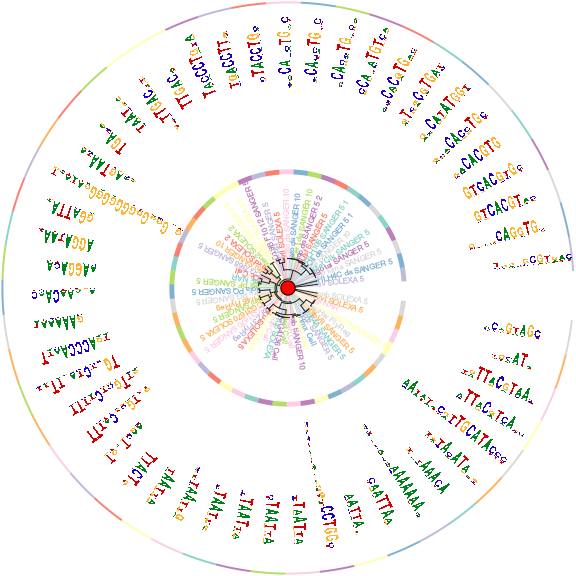## 3.5 plot a sequence logo cloud We can also plot a sequence logo cloud for DNA sequence logo. ## assign groups for motifs groups <- rep(paste("group",1:5,sep=""), each=10) names(groups) <- names(pfms) ## assign group colors group.col <- brewer.pal(5, "Set3") names(group.col)<-paste("group",1:5,sep="") ## use MotIV to calculate the distances of motifs jaspar.scores <- MotIV::readDBScores(file.path(find.package("MotIV"), "extdata", "jaspar2010_PCC_SWU.scores")) d <- MotIV::motifDistances(lapply(pfms, pfm2pwm)) hc <- MotIV::motifHclust(d, method="average") ## convert the hclust to phylog object phylog <- hclust2phylog(hc) ## reorder the pfms by the order of hclust leaves <- names(phylog$leaves)
pfms <- pfms[leaves]
## create a list of pfm objects
pfms <- lapply(names(pfms), function(.ele, pfms){
new("pfm",mat=pfms[[.ele]], name=.ele)}
,pfms)
## extract the motif signatures
motifSig <- motifSignature(pfms, phylog, groupDistance=0.01, min.freq=1)
## draw the motifs with a tag-cloud style.
motifCloud(motifSig, scale=c(6, .5),
layout="rectangles",
group.col=group.col,
groups=groups,
draw.legend=TRUE)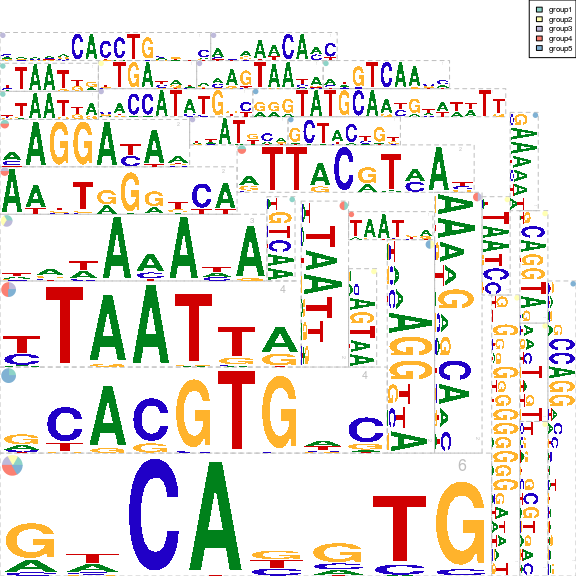## 3.7 motifCircos

We can also plot it with circos style. In circos style, we can plot two group of motifs and with multiple color rings.

## plot the logo stack with radial style.
motifCircos(phylog=phylog, pfms=pfms, pfms2=sig,
col.tree.bg=rep(color, each=5), col.tree.bg.alpha=0.3,
col.leaves=rep(rev(color), each=5),
col.inner.label.circle=gpCol,
inner.label.circle.width=0.03,
col.outer.label.circle=gpCol,
outer.label.circle.width=0.03,
r.rings=c(0.02, 0.03, 0.04),
col.rings=list(sample(colors(), 50),
sample(colors(), 50),
sample(colors(), 50)),
angle=350, motifScale="logarithmic")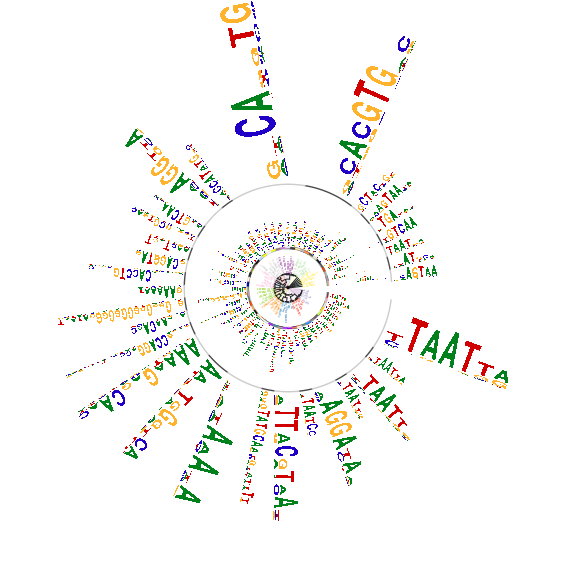## 3.8 motifPiles

We can also plot it with pile style. In pile style, we can plot two group of motifs and with multiple color annoations.

## plot the logo stack with radial style.
motifPiles(phylog=phylog, pfms=pfms, pfms2=sig,
col.tree=rep(color, each=5),
col.leaves=rep(rev(color), each=5),
col.pfms2=gpCol,
r.anno=c(0.02, 0.03, 0.04),
col.anno=list(sample(colors(), 50),
sample(colors(), 50),
sample(colors(), 50)),
motifScale="logarithmic",
plotIndex=TRUE,
groupDistance=0.01)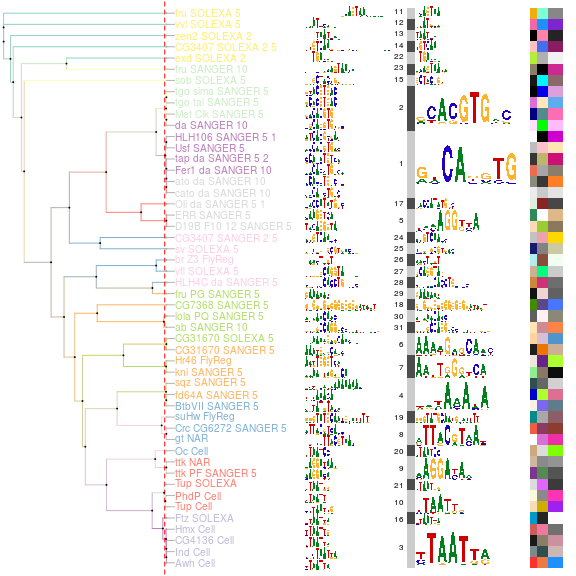# 4 docker container for motifStack}

allows software to be packaged into containers and the containers can be run any platform as well using a virtual machine called boot2docker. motifStack has its docker image stored in . Users can download the image and run.

docker pull jianhong/motifstack_1.13.6
mkdir tmp4motifstack ## this will be the share folder for your host and container.
docker run -ti --rm -v {PWD}/tmp4motifstack:/volume/data jianhong/motifstack_1.13.6 R ## in R setwd("/tmp") library(motifStack) packageVersion("motifStack") pcmpath <- "pcmsDatasetFly" pcms <- readPCM(pcmpath) pfms <- lapply(pcms, pcm2pfm) matalign_path <- "/usr/bin/matalign" neighbor_path <- "/usr/bin/phylip/neighbor" outpath <- "output" system(paste("perl MatAlign2tree.pl --in . --pcmpath", pcmpath, "--out", outpath, "--matalign", matalign_path, "--neighbor", neighbor_path, "--tree","UPGMA")) newickstrUPGMA <- readLines(con=file.path(outpath, "NJ.matalign.distMX.nwk")) phylog <- newick2phylog(newickstrUPGMA, FALSE) leaves <- names(phylogleaves)
motifs <- pfms[leaves]
motifSig <- motifSignature(motifs, phylog, groupDistance=2, min.freq=1, trim=.2)
sig <- signatures(motifSig)
gpCol <- sigColor(motifSig)
leaveNames <- gsub("^Dm_", "", leaves)
pdf("/volume/data/test.pdf", width=8, height=11)
motifPiles(phylog=phylog, DNAmotifAlignment(motifs), sig,
col.pfms=gpCol, col.pfms.width=.01,
col.pfms2=gpCol, col.pfms2.width=.01,
labels.leaves=leaveNames,
plotIndex=c(FALSE, TRUE), IndexCex=1,
groupDistance=2, clabel.leaves=1)
dev.off()


You will see the test.pdf file in the folder of tmp4motifstack.

# 5 Session Info

sessionInfo()
## R version 3.3.1 (2016-06-21)
## Platform: x86_64-pc-linux-gnu (64-bit)
## Running under: Ubuntu 16.04.1 LTS
##
## locale:
##   LC_CTYPE=en_US.UTF-8       LC_NUMERIC=C
##   LC_TIME=en_US.UTF-8        LC_COLLATE=C
##   LC_MONETARY=en_US.UTF-8    LC_MESSAGES=en_US.UTF-8
##   LC_PAPER=en_US.UTF-8       LC_NAME=C
##  LC_MEASUREMENT=en_US.UTF-8 LC_IDENTIFICATION=C
##
## attached base packages:
##   stats4    parallel  grid      stats     graphics  grDevices utils
##   datasets  methods   base
##
## other attached packages:
##   RColorBrewer_1.1-2  MotifDb_1.16.0      motifStack_1.18.0
##   Biostrings_2.42.0   XVector_0.14.0      IRanges_2.8.0
##  BiocGenerics_0.20.0 grImport_0.9-0      XML_3.98-1.4
##  BiocStyle_2.2.0
##
## loaded via a namespace (and not attached):
##   Rcpp_0.12.7                plyr_1.8.4
##   formatR_1.4                GenomeInfoDb_1.10.0
##   bitops_1.0-6               tools_3.3.1
##   zlibbioc_1.20.0            digest_0.6.10
##   evaluate_0.10              tibble_1.2
##  lattice_0.20-34            BSgenome_1.42.0
##  Matrix_1.2-7.1             yaml_2.1.13
##  seqLogo_1.40.0             rtracklayer_1.34.0
##  stringr_1.1.0              knitr_1.14
##  Biobase_2.34.0             BiocParallel_1.8.0
##  munsell_0.4.3              RCurl_1.95-4.8